Courses

# EM Waves in Dielectrics and Conductors - Electromagnetic Theory, CSIR-NET Physical Sciences Physics Notes | EduRev

## Physics for IIT JAM, UGC - NET, CSIR NET

Created by: Akhilesh Thakur

## Physics : EM Waves in Dielectrics and Conductors - Electromagnetic Theory, CSIR-NET Physical Sciences Physics Notes | EduRev

The document EM Waves in Dielectrics and Conductors - Electromagnetic Theory, CSIR-NET Physical Sciences Physics Notes | EduRev is a part of the Physics Course Physics for IIT JAM, UGC - NET, CSIR NET.
All you need of Physics at this link: Physics

In this lecture you will learn:

• Wave propagation in dielectric media
• Waves propagation in conductive media

Review: Plane Waves in Free Space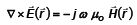Ampere’s Law: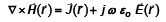Complex Wave Equation: Assume: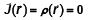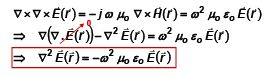For a plane wave in free space we know the E-field and H-field phasors to be: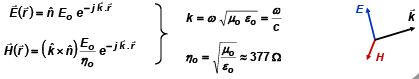Waves in a Dielectric Medium – Wave Equation

Suppose we have a plane wave of the form,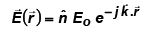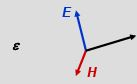traveling in an infinite dielectric medium with permittivity ε

What is different from wave propagation in free space?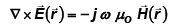Ampere’s Law: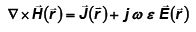Complex Wave Equation: Assume: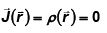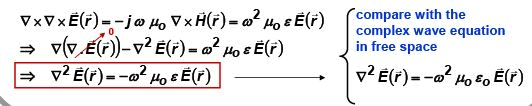Waves in a Dielectric Medium – Dispersion Relation

Substitute the plane wave solution: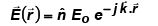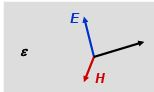in the complex wave equation: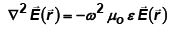To get: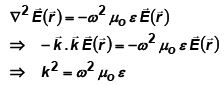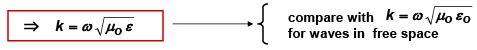Refractive Index:

Define refractive index “n” of a dielectric medium as: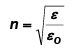Waves in a Dielectric Medium – Velocity

Plane wave: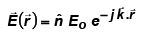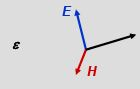Dispersion relation: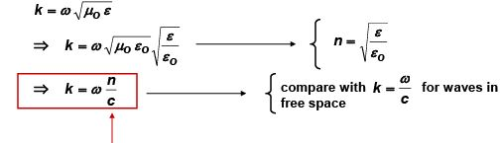The velocity of waves in a dielectric medium is reduced from the velocity of waves in free space by the refractive index

• Velocity of waves in free space: c
• Velocity of waves in dielectric medium of refractive index n: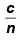Waves in a Dielectric Medium - Wavelength

Plane wave in a dielectric medium: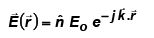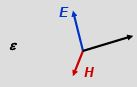Dispersion relation: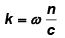But the magnitude of the wavevector is related to the wavelength by the relation: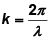So for a dielectric medium we get: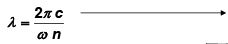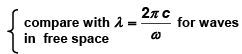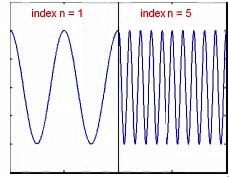The wavelength of plane waves in a dielectric medium is reduced from the wavelength of plane waves of the same frequency in free space by the refractive index

Waves in a Dielectric Medium – Magnetic Field

Plane wave: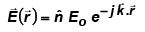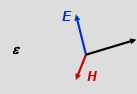Calculate the magnetic field: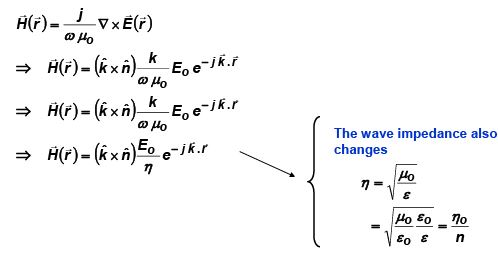Waves in a Conductive Medium – Complex Permittivity

Suppose we have a plane wave of the form,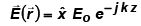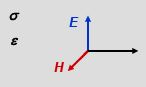traveling in an infinite medium with conductivity σ and permittivity ε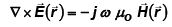Complex Wave Equation: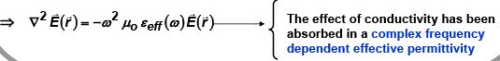Waves in a Conductive Medium – Complex Refractive Index

Plane wave: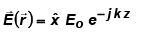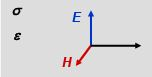Dispersion relation: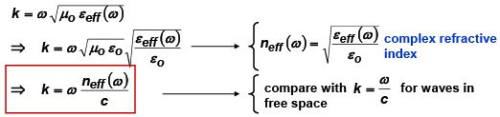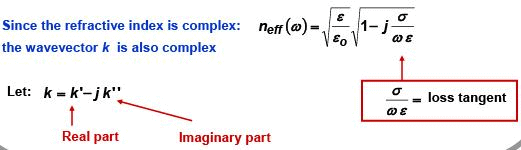Waves in a Conductive Medium – Complex Wavevector

Plane wave: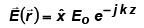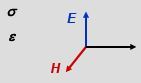Complex wavevector: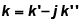What are the implications of a complex wavevector?

• Wave decays exponentially with distance as it propagates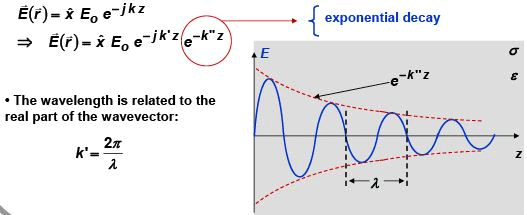Waves in a Conductive Medium – Magnetic Field

Plane wave: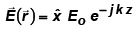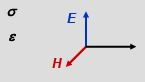Calculate the magnetic field: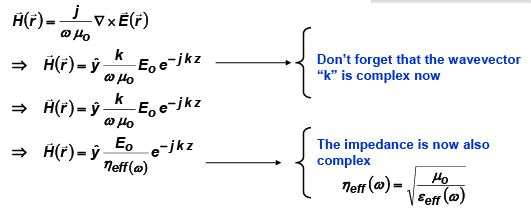Note: The E-field and the H-field are no longer in phase since  ηeff(ω )  is complex

Waves in a Conductive Medium – Power Flow

Plane wave: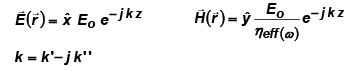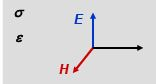Note that: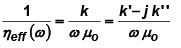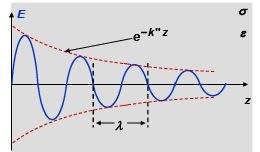Poynting vector and time average power per unit area: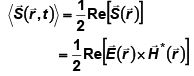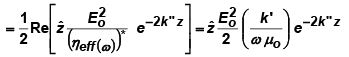Time average power per unit area decays exponentially with distance because energy is dissipated in a conductive medium due to I2R (or J.E) type of losses and this energy dissipated is taken away from the plane wave

Loss Tangent and Dielectric Relaxation Time - I

The complex wavevector is: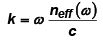The complex refractive index is: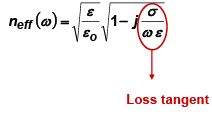Loss tangent =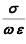But the dielectric relaxation time was: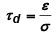⇒ Loss tangent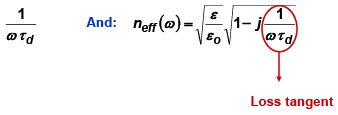Loss Tangent and Dielectric Relaxation Time - II

There are two possible scenarios:

High frequency and/or low conductivity case (e.g. lossy dielectrics)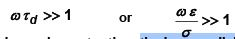The frequency is much greater than the inverse dielectric relaxation time

⇒The conductive medium does not have enough time to react to the electromagnetic wave

⇒No appreciable currents flow in the conductive medium

Low frequency and/or high conductivity case (e.g. Imperfect metals)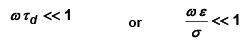The frequency is much smaller than the inverse dielectric relaxation time

⇒The conductive medium has enough time to react to the electromagnetic wave

⇒Appreciable currents flow in the conductive medium

Waves in a Conductive Medium – Lossy Dielectrics

Plane waves: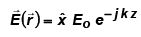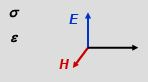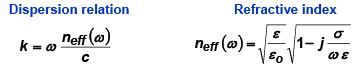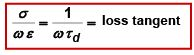Lossy dielectric approximation: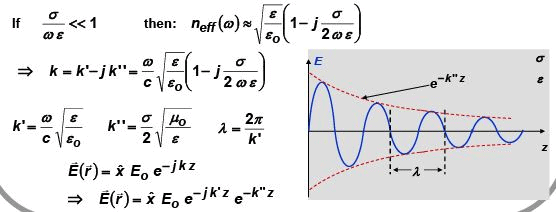Waves in a Conductive Medium – Imperfect Metals

Now consider the case when: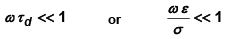The frequency is much smaller than the inverse dielectric relaxation time

⇒The conductive medium has enough time to react to the electromagnetic wave

⇒Appreciable currents flow in the conductive medium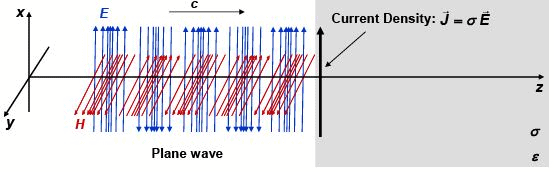These currents try to screen out the magnetic field and, therefore, prevent the electromagnetic wave from going into the conductor

Waves in a Conductive Medium – Imperfect Metals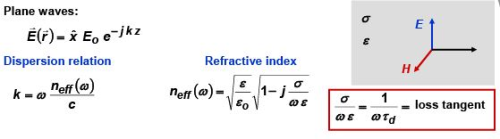Imperfect metal approximation: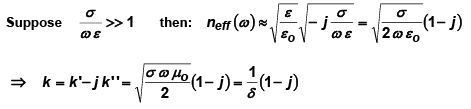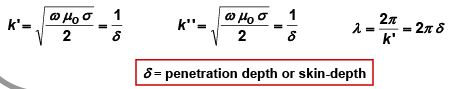Waves in a Conductive Medium – Imperfect Metals

Due to current screening the wave decays within a few skin-depths: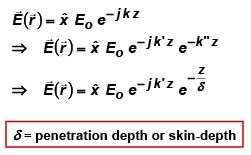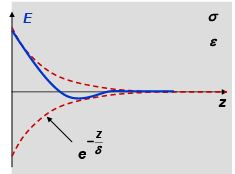Since the wavelength λ inside the medium is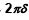, the wave hardly propagates one wavelength distance into the medium
The screening current density, given by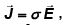is non-zero only in a layer of thickness equal to skin-depth δ near the surface

Waves in a Conductive Medium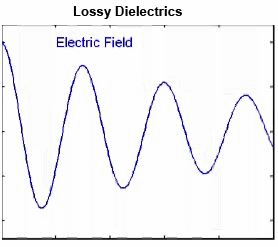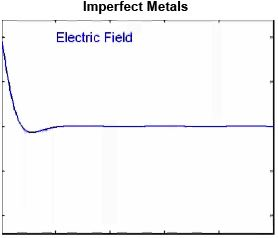159 docs

,

,

,

,

,

,

,

,

,

,

,

,

,

,

,

,

,

,

,

,

,

,

,

,

;